Parents' Night Out

Thursday, September 27
7:00 pm
Mia Figlia Restaurant
5304 West Devon Avenue

Join us for a Parents' Night Out at Mia Figlia Restaurant. There will be complimentary appetizers (brushetta, pizza, calamari) and a cash bar. Catch up with old friends or make new ones! Please sign up HERE so we have a head count to give the restaurant. Mia Figlia customers can park in the Edgebrook Lutheran Church parking lot by the restaurant or on the street.

 table div table+table+table div table{width:100%;padding:0}table div table+table+table div table img{width:96.23%;padding:0;float:none}table div table+table+table div table td{width:100%;padding:0 1.88% 18px}/* styles */Friends of Northside Meeting

Tuesday, September 25
7:00 pm
Faculty Lounge

Learn more about what's happening at NCP by attending our Friends of Northside meeting. All are welcome!

 table div table+table+table+table+table div table{width:100%;padding:0}table div table+table+table+table+table div table img{width:96.23%;padding:0;float:none}table div table+table+table+table+table div table td{width:100%;padding:0 1.88% 18px}/* styles */Parking Lot Safety

Parents, please remember that ALL VEHICLES MUST EXIT ONTO KEDZIE. You may enter the parking lot from Kedzie or Bryn Mawr, but regardless of where you enter, all cars must exit onto Kedzie. For the safety of our students and the rest of the Northside community, please do not exit onto Bryn Mawr.

 table div table+table+table+table+table+table+table div table{width:100%;padding:0}table div table+table+table+table+table+table+table div table img{width:96.23%;padding:0;float:none}table div table+table+table+table+table+table+table div table td{width:100%;padding:0 1.88% 18px}/* styles */Upcoming Volunteer OpportunitiesAnnual Appeal Mailing

Thursday, October 4
4:15 - 6:15 pm
Faculty Lounge

We're kicking off our Annual Appeal and need folks to label and stuff envelopes for the mailing that goes to all NCP families and friends. No skills required and guaranteed to be a good time! To sign up, click HERE.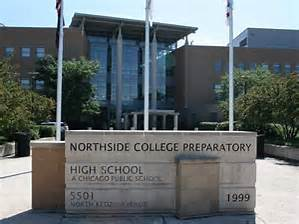Northside's Selective Enrollment Open House

Sunday, October 20
11:00 am - 2:00 pm

We need 100 volunteers to work this busy but super-fun event! Parents help answer questions and direct crowds in the gym, outside and all over the building.

Sign up HERE to donate snacks for the students.

 table div table+table+table+table+table+table+table+table+table+table+table+table div table{width:100%;padding:0}table div table+table+table+table+table+table+table+table+table+table+table+table div table img{width:96.23%;padding:0;float:none}table div table+table+table+table+table+table+table+table+table+table+table+table div table td{width:100%;padding:0 1.88% 18px}/* styles */Volunteers Still Needed

Make a commitment to Northside by offering your time to work with other volunteers in one of these rewarding, on-going positions. Email Friends of Northside to indicate your interest or ask questions.

Eblast Co-Editor

Compose and distribute This Week at Northside and prepare other standalone blasts as requested.

Update and maintain the Friends of Northside Facebook and Twitter accounts.

Graphic Designer

Provide professional guidance and materials for various design needs throughout the year.

Website Editor

Keep the Friends of Northside website current and enhance its visual appeal.

Database Manager

Maintain the Friends of Northside CRM (customer relationship management) database and develop meaningful reports.

Teachers' Lounge Volunteer

Help stock and maintain the Teachers’ Lounge. This is a great low-commitment way to give back to NCP, only 30-45 minutes once a week, you pick the day and time. Call or text Eileen at 617-642-6267 for details or donate goods HERE.

Join the Gala Committee

The Gala is NCP’s major spring fundraiser. Get involved, get to know NCP staff and parents and make a profound impact on Northside College Prep.

When is the Gala?
Friday, March 8, 2019

What’s the time commitment for the team?
Meetings begin at the end of September. The time commitment depends on your role. There is something for everyone. Please join us!

What roles are available?
* Entertainment Chair - one or two people to determine and arrange the entertainment for the Gala.
* Auction Item Solicitors - at least five people to solicit auction items. It requires just a few hours a week and can be done in person or via phone or email.

Email ncpgaladonate@gmail.com for more information or to join the team!

 table div table+table+table+table+table+table+table+table+table+table+table+table+table+table+table div table{width:100%;padding:0}table div table+table+table+table+table+table+table+table+table+table+table+table+table+table+table div table img{width:96.23%;padding:0;float:none}table div table+table+table+table+table+table+table+table+table+table+table+table+table+table+table div table td{width:100%;padding:0 1.88% 18px}/* styles */CPS Volunteer Requirements

According to CPS policy, anybody who is going to be in contact with students must be approved as a CPS volunteer. There are two levels of volunteers, Level 1 and Level 2.

Level II Volunteers

(This process can be completed in a day & is required even of volunteers for Friends of Northside events)
A fingerprint criminal background check is not required for Level II Volunteers.

A Level II Volunteer is:

1. A parent providing supervised volunteer service in their child’s school or classroom for less than 10 hours/week;
2. Any individual providing volunteer service for less than 5 hours/week at a school where s/he does not have a child enrolled;
3. An individual providing incidental volunteer service with no ongoing individualized interaction with a student(s) including those who speak at a class/assembly, judge academic competitions, give musical performance, participate in the “Principal for a Day” program, job shadowing event or other one-time event provided where there is direct supervision of the activity/event by regular school employees;
4. A parent accompanying his/her child’s class on a one-day field trip or another type of extracurricular activity that does not involve an overnight stay;
5. An individual providing volunteer service on projects/activities involving no or nominal contact with children (home-based volunteer activities, volunteers serving at Central or Network office).
 1 A parent providing supervised volunteer service in their child’s school or classroom for less than 10 hours/week;
 2 Any individual providing volunteer service for less than 5 hours/week at a school where s/he does not have a child enrolled;
 3 An individual providing incidental volunteer service with no ongoing individualized interaction with a student(s) including those who speak at a class/assembly, judge academic competitions, give musical performance, participate in the “Principal for a Day” program, job shadowing event or other one-time event provided where there is direct supervision of the activity/event by regular school employees;
 4 A parent accompanying his/her child’s class on a one-day field trip or another type of extracurricular activity that does not involve an overnight stay;
 5 An individual providing volunteer service on projects/activities involving no or nominal contact with children (home-based volunteer activities, volunteers serving at Central or Network office).

Level I Volunteers

(Please allow 8-12 weeks for the entire process)
A fingerprint criminal background check is required for all Level I Volunteers.

A Level I volunteer is:

1. A parent providing volunteer service in their child’s school or classroom for 10 or more hours/week;
2. An individual providing volunteer service for 5 or more hours/week at a school where s/he does not have a child enrolled;
3. Any individual chaperoning an overnight school-sponsored trip, regardless of the hours/week that the volunteer serves (See also the Student Travel Policy).
 1 A parent providing volunteer service in their child’s school or classroom for 10 or more hours/week;
 2 An individual providing volunteer service for 5 or more hours/week at a school where s/he does not have a child enrolled;
 3 Any individual chaperoning an overnight school-sponsored trip, regardless of the hours/week that the volunteer serves (See also the Student Travel Policy).

To become a volunteer, please visit CPS' Volunteer website and follow the instructions. There is also a link on Northside's website on the Parents page Northside Volunteers.

Assistant Principal, Kaitlin O'Hare is Northside's Volunteer Coordinator. After applying to become a volunteer, you must visit the school and show Ms. O'Hare your ID, so she can approve you to move forward in the volunteer process. For Level II volunteers, that is the only step you must take. For Level 1, you will receive further instructions from CPS.

 table div table+table+table+table+table+table+table+table+table+table+table+table+table+table+table+table+table div table{width:100%;padding:0}table div table+table+table+table+table+table+table+table+table+table+table+table+table+table+table+table+table div table img{width:96.23%;padding:0;float:none}table div table+table+table+table+table+table+table+table+table+table+table+table+table+table+table+table+table div table td{width:100%;padding:0 1.88% 18px}/* styles */This Week in Sports

Come to a game or match and support our student athletes!

Womens' Tennis

▪ Monday, September 24, 5:00 pm vs Taft at Peterson Park (V)
 ▪ Monday, September 24, 5:00 pm vs Taft at Peterson Park (V)

Mens' Softball

▪ Monday, September 24, 4:30 pm vs Taft at Taft (V)
▪ Tuesday, September 25, 4:30 pm vs. Steinmetz at Legion Park (V)
▪ Wednesday, September 26, 4:30 pm vs. Payton College Prep at Legion Park (V)
 ▪ Monday, September 24, 4:30 pm vs Taft at Taft (V)
 ▪ Tuesday, September 25, 4:30 pm vs. Steinmetz at Legion Park (V)
 ▪ Wednesday, September 26, 4:30 pm vs. Payton College Prep at Legion Park (V)

Womens' Golf

▪ Monday, September 24, 4:30 pm vs Whitney Young at Billy Caldwell
▪ Wednesday, September 26, 12:00 pm, Playoff for City Championship at Billy Caldwell
 ▪ Monday, September 24, 4:30 pm vs Whitney Young at Billy Caldwell
 ▪ Wednesday, September 26, 12:00 pm, Playoff for City Championship at Billy Caldwell

Mens' Soccer

▪ Tuesday, September 25, 7:00 pm vs Kelly HS at Kelly HS (V)
 ▪ Tuesday, September 25, 7:00 pm vs Kelly HS at Kelly HS (V)

Womens' Volleyball

▪ Tuesday, September 25, 5:00 (V) and 6:00 (SO) pm vs Lane Tech at NCP
▪ Saturday, September 29, time TBD, NCP Varsity Invite in the NCP Gym (V)
▪ Saturday, September 29, 9:45 am vs Alcott at Amundsen HS (F)
▪ Saturday, September 29, 10:30 am vs Steinmetz at Amundsen HS (F)
 ▪ Tuesday, September 25, 5:00 (V) and 6:00 (SO) pm vs Lane Tech at NCP
 ▪ Saturday, September 29, time TBD, NCP Varsity Invite in the NCP Gym (V)
 ▪ Saturday, September 29, 9:45 am vs Alcott at Amundsen HS (F)
 ▪ Saturday, September 29, 10:30 am vs Steinmetz at Amundsen HS (F)

Girls' Swimming

▪ Monday, September 24, 5:00 pm vs. Lincoln Park HS at Lincoln Park HS (V)
 ▪ Monday, September 24, 5:00 pm vs. Lincoln Park HS at Lincoln Park HS (V)
 /* styles */ Visit the NCP Athletics website for additional information about Northside teams, and download the 8 to 18 app via iTunes or Android to follow them on your phone.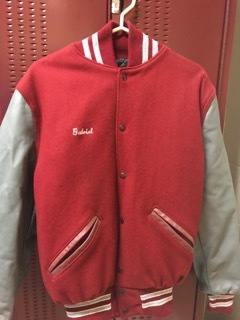Order Your Northside Athletic Jacket Now

If you're interested in purchasing an athletic jacket, please see Mr. Pagani in the gym for an order form or click HERE to download a copy.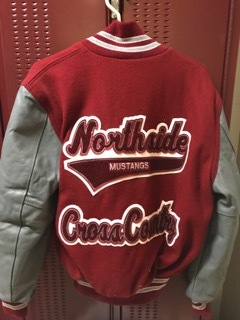Contact Mr. Pagani with any questions.

Deadline to order: Wednesday, October 10

 table div table+table+table+table+table+table+table+table+table+table+table+table+table+table+table+table+table+table+table+table+table+table div table{width:100%;padding:0}table div table+table+table+table+table+table+table+table+table+table+table+table+table+table+table+table+table+table+table+table+table+table div table img{width:96.23%;padding:0;float:none}table div table+table+table+table+table+table+table+table+table+table+table+table+table+table+table+table+table+table+table+table+table+table div table td{width:100%;padding:0 1.88% 18px}/* styles */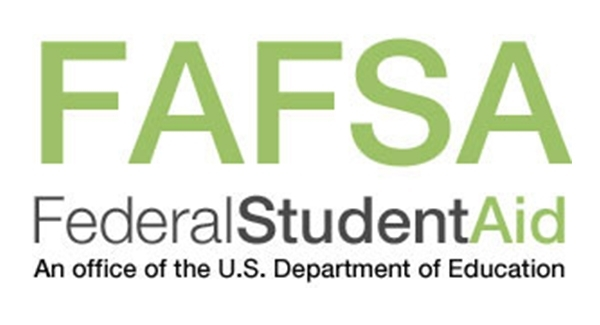FAFSA Completion Workshop

Presented by Ladder Up

Tuesday, October 16
6:00 - 8:00 pm
NCP Library, 2nd Floor

The FAFSA form should be filled out as soon as possible because it helps to determine your eligibility for federal, state, local and college scholarships, grants and loans. If you have not completed your FAFSA form or have questions, this workshop will provide students and parents support with their financial aid forms.

For students attending college in the 2019-2020 school year, you will need financial information from 2017.

· Your Social Security card. It is important that you enter your Social Security Number correctly!

· Your (and if married, your spouse's) 2017 federal income tax return and any other records so that you can prepare your FAFSA carefully and correctly

Contact Mr. Alexander Hughes in the counseling office if you have any questions. He can be reached at 773-534-3974 or ACHughes2@cps.edu.

 table div table+table+table+table+table+table+table+table+table+table+table+table+table+table+table+table+table+table+table+table+table+table+table+table div table{width:100%;padding:0}table div table+table+table+table+table+table+table+table+table+table+table+table+table+table+table+table+table+table+table+table+table+table+table+table div table img{width:96.23%;padding:0;float:none}table div table+table+table+table+table+table+table+table+table+table+table+table+table+table+table+table+table+table+table+table+table+table+table+table div table td{width:100%;padding:0 1.88% 18px}/* styles */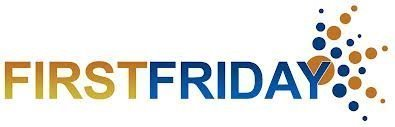/* styles */ Friday, October 5 8:00 - 10:00 am Panera Bread, 6059 N Lincoln Ave Friends of Northside gathers on the First Friday morning of every month for coffee and conversation. This casual get-together gives you a chance to meet and network with other parents. Feel free to come and go as it suits your schedule.
 table div table+table+table+table+table+table+table+table+table+table+table+table+table+table+table+table+table+table+table+table+table+table+table+table+table+table+table div table{width:100%;padding:0}table div table+table+table+table+table+table+table+table+table+table+table+table+table+table+table+table+table+table+table+table+table+table+table+table+table+table+table div table img{width:96.23%;padding:0;float:none}table div table+table+table+table+table+table+table+table+table+table+table+table+table+table+table+table+table+table+table+table+table+table+table+table+table+table+table div table td{width:100%;padding:0 1.88% 18px}/* styles */No School

Columbus Day
Monday, October 8

 table div table+table+table+table+table+table+table+table+table+table+table+table+table+table+table+table+table+table+table+table+table+table+table+table+table+table+table+table+table div table{width:100%;padding:0}table div table+table+table+table+table+table+table+table+table+table+table+table+table+table+table+table+table+table+table+table+table+table+table+table+table+table+table+table+table div table img{width:96.23%;padding:0;float:none}table div table+table+table+table+table+table+table+table+table+table+table+table+table+table+table+table+table+table+table+table+table+table+table+table+table+table+table+table+table div table td{width:100%;padding:0 1.88% 18px}/* styles */Other Important October Dates

Friday, October 5--First Friday
Monday, October 8--Columbus Day, no school
Thursday, October 11--Athletic Boosters Meeting
Tuesday, October 16--LSC Meeting
Tuesday, October 16--FAFSA Completion Workshop
Saturday, October 20--NCP Open House
Tuesday, October 23--FON Meeting
Thursday, October 25--Halloween Concert
Friday, October 26--Halloween Dance sponsored by the Latin Club

 table div table+table+table+table+table+table+table+table+table+table+table+table+table+table+table+table+table+table+table+table+table+table+table+table+table+table+table+table+table+table+table div table{width:100%;padding:0}table div table+table+table+table+table+table+table+table+table+table+table+table+table+table+table+table+table+table+table+table+table+table+table+table+table+table+table+table+table+table+table div table img{width:96.23%;padding:0;float:none}table div table+table+table+table+table+table+table+table+table+table+table+table+table+table+table+table+table+table+table+table+table+table+table+table+table+table+table+table+table+table+table div table td{width:100%;padding:0 1.88% 18px}/* styles */table.module-31{width:94.34%;padding:0}table div table+table+table+table+table+table+table+table+table+table+table+table+table+table+table+table+table+table+table+table+table+table+table+table+table+table+table+table+table+table+table+table div table{width:94.34%;float:none;margin-left:auto;margin-right:auto;padding:0}table div table+table+table+table+table+table+table+table+table+table+table+table+table+table+table+table+table+table+table+table+table+table+table+table+table+table+table+table+table+table+table+table div table a{border:0 none;text-decoration:none}table div table+table+table+table+table+table+table+table+table+table+table+table+table+table+table+table+table+table+table+table+table+table+table+table+table+table+table+table+table+table+table+table div table img{width:100%!important;border:0 none;text-decoration:none}table div table+table+table+table+table+table+table+table+table+table+table+table+table+table+table+table+table+table+table+table+table+table+table+table+table+table+table+table+table+table+table+table div table td{width:100%;padding:0}/* styles */
 /* styles */ Check out The HoofBeat, Northside's online newspaper, to get NCP news from the student perspective!
 table div table+table+table+table+table+table+table+table+table+table+table+table+table+table+table+table+table+table+table+table+table+table+table+table+table+table+table+table+table+table+table+table+table+table div table{width:100%;padding:0}table div table+table+table+table+table+table+table+table+table+table+table+table+table+table+table+table+table+table+table+table+table+table+table+table+table+table+table+table+table+table+table+table+table+table div table img{width:96.23%;padding:0;float:none}table div table+table+table+table+table+table+table+table+table+table+table+table+table+table+table+table+table+table+table+table+table+table+table+table+table+table+table+table+table+table+table+table+table+table div table td{width:100%;padding:0 1.88% 18px}/* styles */table.module-34{width:57.17%;padding:0}table div table+table+table+table+table+table+table+table+table+table+table+table+table+table+table+table+table+table+table+table+table+table+table+table+table+table+table+table+table+table+table+table+table+table+table div table{width:57.17%;float:none;margin-left:auto;margin-right:auto;padding:0}table div table+table+table+table+table+table+table+table+table+table+table+table+table+table+table+table+table+table+table+table+table+table+table+table+table+table+table+table+table+table+table+table+table+table+table div table a{border:0 none;text-decoration:none}table div table+table+table+table+table+table+table+table+table+table+table+table+table+table+table+table+table+table+table+table+table+table+table+table+table+table+table+table+table+table+table+table+table+table+table div table img{width:100%!important;border:0 none;text-decoration:none}table div table+table+table+table+table+table+table+table+table+table+table+table+table+table+table+table+table+table+table+table+table+table+table+table+table+table+table+table+table+table+table+table+table+table+table div table td{width:100%;padding:0}/* styles */

What is FAN?

FAN is Family Action Network, an organization which connects parents, educators and professionals through collaborative programming that educates, inspires and positively impacts the broader community. Nearly every week during the school year there will be a dynamic and important speaker, discussing the issues that interest you.

FAN events are free to all.

The Class: A Life-Changing Teacher, His World-Changing Kids, and the Most Inventive Classroom in America

Heather Won Tesoriero
Emmy-winning former producer for CBS News

Monday, September 24
12:00 pm, Loyola University Chicago School of Law
25. E. Pearson St., Chicago
RSVP HERE (required)
7:00 pm, New Trier High School/Northfield, Cornog Auditorium
7 Happ Rd., Northfield

How to be a Happier Parent: Raising a Family, Having a Life, and Loving (Almost) Every Minute

KJ Dell'Antonia
Writer and regular contributor to The New York Times, and former editor of its Motherlode blog, 2011-16.

Friday, September 28, 7:00 pm
New Trier High School/Northfield, Cornog Auditorium
7 Happ Rd., Northfield

One Person, No Vote: Examining the History of Voter Suppression

Carol Anderson, Ph.D.
Charles Howard Candler Professor and Chair of African American Studies, Emory University

Monday, October 1, 7:00 pm
Evanston Township High School Auditorium
1600 Dodge Ave., Evanston

 table div table+table+table+table+table+table+table+table+table+table+table+table+table+table+table+table+table+table+table+table+table+table+table+table+table+table+table+table+table+table+table+table+table+table+table+table+table div table{width:100%;padding:0}table div table+table+table+table+table+table+table+table+table+table+table+table+table+table+table+table+table+table+table+table+table+table+table+table+table+table+table+table+table+table+table+table+table+table+table+table+table div table img{width:96.23%;padding:0;float:none}table div table+table+table+table+table+table+table+table+table+table+table+table+table+table+table+table+table+table+table+table+table+table+table+table+table+table+table+table+table+table+table+table+table+table+table+table+table div table td{width:100%;padding:0 1.88% 18px}/* styles */FON Connect

Friends of Northside uses a customer/constituent relationship management (CRM) system known as FON Connect that allows our parent volunteer organizations to work from one database. It is not a CPS database and is completely different and separate from the CPS Parent Portal and Northside's EPay website.

FON Connect serves as the NCP Family Directory and allows users to review their donation history, update their contact information, register for upcoming events and more. Each parent has a separate account. Transactions, including donations, are recorded in the account of the parent who initiated the transaction or donation.

If you’re new to FON Connect, you'll need to set up a login and password. To do so:

▪ Click on “Forgot Password?”
▪ Check your email for the link to create a login name and password.
 ▪ Click on “Forgot Password?”
 ▪ Check your email for the link to create a login name and password.

Once you have set up your FON Connect account, keep your login and password in a safe, convenient place because they will be your keys to the database throughout your years at Northside.

 table div table+table+table+table+table+table+table+table+table+table+table+table+table+table+table+table+table+table+table+table+table+table+table+table+table+table+table+table+table+table+table+table+table+table+table+table+table+table+table div table{width:100%;padding:0}table div table+table+table+table+table+table+table+table+table+table+table+table+table+table+table+table+table+table+table+table+table+table+table+table+table+table+table+table+table+table+table+table+table+table+table+table+table+table+table div table img{width:96.23%;padding:0;float:none}table div table+table+table+table+table+table+table+table+table+table+table+table+table+table+table+table+table+table+table+table+table+table+table+table+table+table+table+table+table+table+table+table+table+table+table+table+table+table+table div table td{width:100%;padding:0 1.88% 18px}/* styles */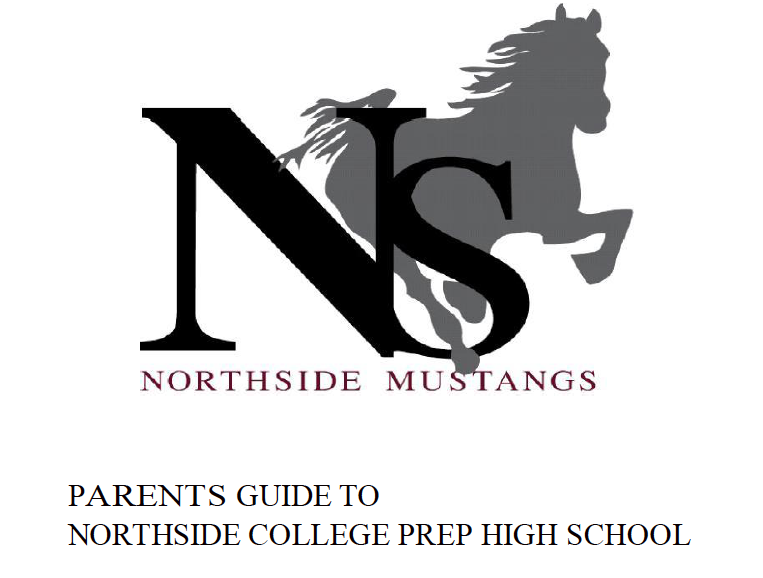Navigating Northside

Navigating Northside, our parent handbook, is available on the school website under the Parents tab. Click HERE to access it directly.

 table div table+table+table+table+table+table+table+table+table+table+table+table+table+table+table+table+table+table+table+table+table+table+table+table+table+table+table+table+table+table+table+table+table+table+table+table+table+table+table+table+table div table{width:100%;padding:0}table div table+table+table+table+table+table+table+table+table+table+table+table+table+table+table+table+table+table+table+table+table+table+table+table+table+table+table+table+table+table+table+table+table+table+table+table+table+table+table+table+table div table img{width:96.23%;padding:0;float:none}table div table+table+table+table+table+table+table+table+table+table+table+table+table+table+table+table+table+table+table+table+table+table+table+table+table+table+table+table+table+table+table+table+table+table+table+table+table+table+table+table+table div table td{width:100%;padding:0 1.88% 18px}/* styles */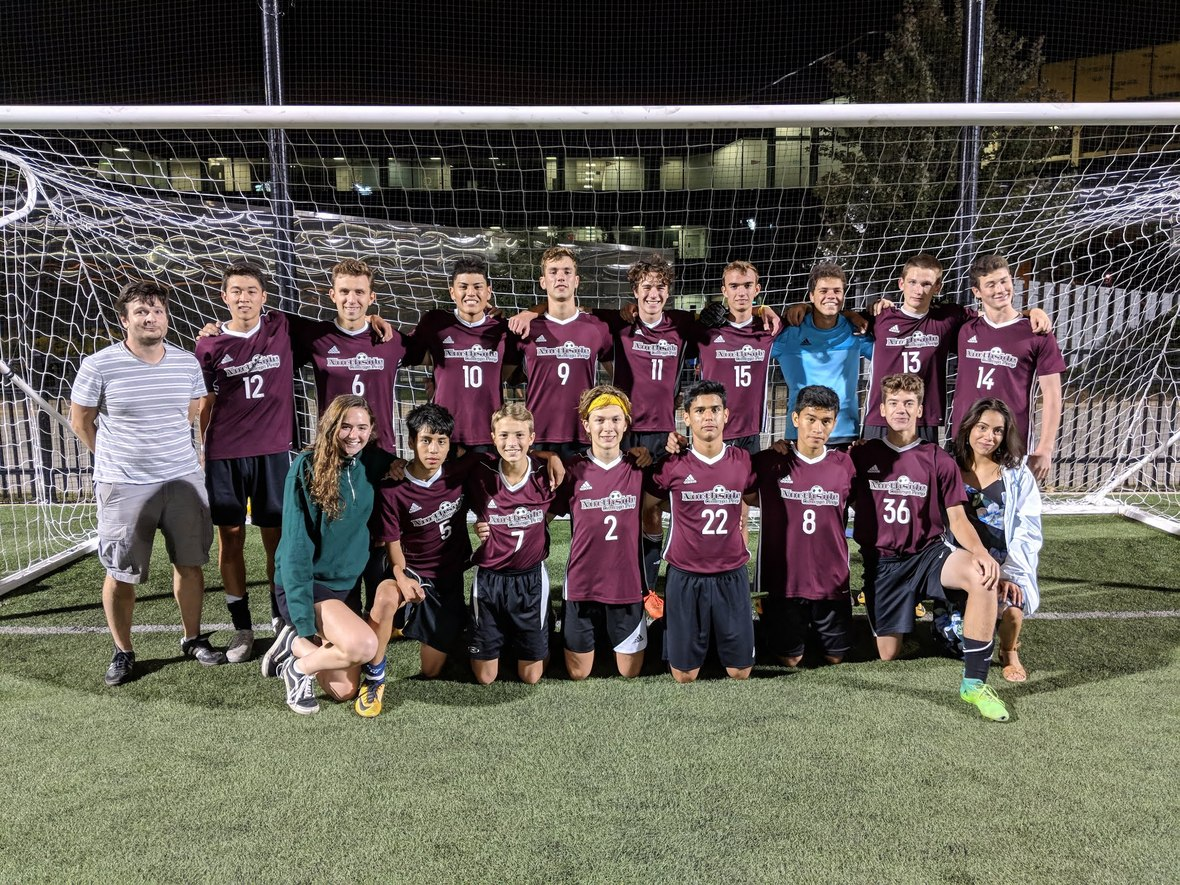NCP Men's Soccer Wins Second Consecutive Conference Title

Needing to score four goals to clinch the conference title the Northside boys soccer team went on a tear last Monday and defeated Schurz 10-1 to clinch their second consecutive conference championship in a row. Congratulations!

 table div table+table+table+table+table+table+table+table+table+table+table+table+table+table+table+table+table+table+table+table+table+table+table+table+table+table+table+table+table+table+table+table+table+table+table+table+table+table+table+table+table+table+table+table+table div table{width:100%;padding:0}table div table+table+table+table+table+table+table+table+table+table+table+table+table+table+table+table+table+table+table+table+table+table+table+table+table+table+table+table+table+table+table+table+table+table+table+table+table+table+table+table+table+table+table+table+table div table img{width:96.23%;padding:0;float:none}table div table+table+table+table+table+table+table+table+table+table+table+table+table+table+table+table+table+table+table+table+table+table+table+table+table+table+table+table+table+table+table+table+table+table+table+table+table+table+table+table+table+table+table+table+table div table td{width:100%;padding:0 1.88% 18px}/* styles */National Association for College Admission Counseling (NACAC) College Fair

Saturday, September 29, 11:00 am – 3:00 pm
McCormick Place
2301 S King Dr.

Free and open to the public, National College Fairs allow students to interact with admission representatives from a wide range of post-secondary institutions.

Click HERE for more information, to see a list of participating school and to register.

Yale Information Sessions

An Associate Director of Undergraduate Admissions will discuss academic programs, residential colleges and campus life, admissions, and financial aid. Free to attend and all are welcome. Registration is recommended but not required.

Thursday, October 4, 06:30 - 08:00 pm
Niles West High School
5701 Oakton St, Skokie
Register HERE

Tuesday, October 9, 06:30 - 08:00 pm
Whitney M. Young Magnet High School
211 S Laflin St, Chicago
Register HERE

100 Black Men of Chicago, Inc.'s - 16th Annual College Scholarship Fair

Saturday, October 13, 9:00 am - 2:00 pm
University of Illinois, at Chicago - Pavilion
525 S Racine Ave

Since 2003, the 100BMC has hosted an annual College Scholarship Fair with up to 200 Colleges and Universities interacting with nearly 5000 students, parents and educational partners.

Students can register HERE.

Tufts University Admissions: Who Gets In and Why

Sunday, October 14, 02:00 - 04:00 pm
DoubleTree by Hilton Hotel Chicago - North Shore Conference Center
9599 Skokie Blvd, Skokie

The program will highlight the following:
1. Academics and campus life at Tufts University
2. The different components of a college application and how those materials are evaluated by selective admissions offices
3. Tips on successful essay writing and the impact of an application supplement
4. The role of recommendations and interviews in the process
5. A case study designed to resemble the admissions process, featuring five real applications from prior Tufts applicants

Students can register HERE for the workshop.

Upcoming College Visits at Northside

Monday, September 24
MacMurray College, 10:15 am
University of Missouri St. Louis, 10:15 am
University of Nebraska at Lincoln, 10:15 am
Northern Michigan University, 10:15 am
Purdue University Northwest - Hammond Campus, 10:15 am
Sacred Heart University, 10:15 am
University of Connecticut, 3:15 pm

Tuesday, September 25
MCPHS - Massachusetts College of Pharmacy & Health Sciences, 9:48 am
Western Illinois University, 9:48 am
Drake University, 11:36 am
Keiser University - *Flagship Campus, FL, 12:26 pm
The College of Wooster, 3:15 pm

Wednesday, September 26
Loras College, 10:31 am
California Lutheran University, 11:22 am
University of California, Riverside, 11:22 am
Duke Kunshan University, 3:15 pm

Thursday, September 27
Beloit College, 9:48 am
University of California, Irvine, 9:48 am
Trinity College, 9:48 am
University of Nevada, Las Vegas, 10:38 am
Stevens Institute of Technology, 10:38 am
Rensselaer Polytechnic Institute, 12:26 pm
University of San Francisco, 3:15 pm

Friday, September 28
University of New Orleans, 9:48 am
Scripps College, 9:48 am
Vassar College, 9:48 am
LIU - Post, 9:48 am
Roanoke College, 10:38 am
Rollins College, 11:36 am
University of California - Davis, 11:36 am
Rose-Hulman Institute of Technology, 3:15 pm

The list of college visits is updated frequently. For the most current information, parents of juniors and seniors should log into their parent account for Naviance.

 table div table+table+table+table+table+table+table+table+table+table+table+table+table+table+table+table+table+table+table+table+table+table+table+table+table+table+table+table+table+table+table+table+table+table+table+table+table+table+table+table+table+table+table+table+table+table+table+table+table+table+table+table div table{width:100%;padding:0}table div table+table+table+table+table+table+table+table+table+table+table+table+table+table+table+table+table+table+table+table+table+table+table+table+table+table+table+table+table+table+table+table+table+table+table+table+table+table+table+table+table+table+table+table+table+table+table+table+table+table+table+table div table img{width:96.23%;padding:0;float:none}table div table+table+table+table+table+table+table+table+table+table+table+table+table+table+table+table+table+table+table+table+table+table+table+table+table+table+table+table+table+table+table+table+table+table+table+table+table+table+table+table+table+table+table+table+table+table+table+table+table+table+table+table div table td{width:100%;padding:0 1.88% 18px}/* styles */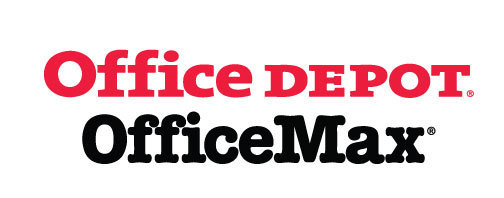Give Back to Northside

Purchase school and office supplies at Office Depot and OfficeMax, and Northside can receive 5% back in credits or FREE supplies. Simply provide our school ID 70031171 at checkout, online or in stores. There’s no cost to you!

 table div table+table+table+table+table+table+table+table+table+table+table+table+table+table+table+table+table+table+table+table+table+table+table+table+table+table+table+table+table+table+table+table+table+table+table+table+table+table+table+table+table+table+table+table+table+table+table+table+table+table+table+table+table+table+table div table{width:100%;padding:0}table div table+table+table+table+table+table+table+table+table+table+table+table+table+table+table+table+table+table+table+table+table+table+table+table+table+table+table+table+table+table+table+table+table+table+table+table+table+table+table+table+table+table+table+table+table+table+table+table+table+table+table+table+table+table+table div table img{width:96.23%;padding:0;float:none}table div table+table+table+table+table+table+table+table+table+table+table+table+table+table+table+table+table+table+table+table+table+table+table+table+table+table+table+table+table+table+table+table+table+table+table+table+table+table+table+table+table+table+table+table+table+table+table+table+table+table+table+table+table+table+table div table td{width:100%;padding:0 1.88% 18px}/* styles */table.module-55{width:66.42%;padding:0}table div table+table+table+table+table+table+table+table+table+table+table+table+table+table+table+table+table+table+table+table+table+table+table+table+table+table+table+table+table+table+table+table+table+table+table+table+table+table+table+table+table+table+table+table+table+table+table+table+table+table+table+table+table+table+table+table div table{width:66.42%;float:none;margin-left:auto;margin-right:auto;padding:0}table div table+table+table+table+table+table+table+table+table+table+table+table+table+table+table+table+table+table+table+table+table+table+table+table+table+table+table+table+table+table+table+table+table+table+table+table+table+table+table+table+table+table+table+table+table+table+table+table+table+table+table+table+table+table+table+table div table a{border:0 none;text-decoration:none}table div table+table+table+table+table+table+table+table+table+table+table+table+table+table+table+table+table+table+table+table+table+table+table+table+table+table+table+table+table+table+table+table+table+table+table+table+table+table+table+table+table+table+table+table+table+table+table+table+table+table+table+table+table+table+table+table div table img{width:100%!important;border:0 none;text-decoration:none}table div table+table+table+table+table+table+table+table+table+table+table+table+table+table+table+table+table+table+table+table+table+table+table+table+table+table+table+table+table+table+table+table+table+table+table+table+table+table+table+table+table+table+table+table+table+table+table+table+table+table+table+table+table+table+table+table div table td{width:100%;padding:0}/* styles */

Support Northside - Shop AmazonSmile

AmazonSmile is a simple and automatic way to support Northside every time you shop.

Please note that our AmazonSmile name is Friends of "North" "Side" (North Side - two words at AmazonSmile).

 table div table+table+table+table+table+table+table+table+table+table+table+table+table+table+table+table+table+table+table+table+table+table+table+table+table+table+table+table+table+table+table+table+table+table+table+table+table+table+table+table+table+table+table+table+table+table+table+table+table+table+table+table+table+table+table+table+table+table div table{width:100%;padding:0}table div table+table+table+table+table+table+table+table+table+table+table+table+table+table+table+table+table+table+table+table+table+table+table+table+table+table+table+table+table+table+table+table+table+table+table+table+table+table+table+table+table+table+table+table+table+table+table+table+table+table+table+table+table+table+table+table+table+table div table img{width:96.23%;padding:0;float:none}table div table+table+table+table+table+table+table+table+table+table+table+table+table+table+table+table+table+table+table+table+table+table+table+table+table+table+table+table+table+table+table+table+table+table+table+table+table+table+table+table+table+table+table+table+table+table+table+table+table+table+table+table+table+table+table+table+table+table div table td{width:100%;padding:0 1.88% 18px}/* styles */Follow Us on Social Media

▪ NCP's Official Facebook Page
▪ Northside Prep Parents Facebook Page, a closed group for parents, by parents, to share their knowledge of Northside
▪ NCP's Twitter Page
▪ Friends of Northside Facebook Page, for news everyone can view
 ▪ Northside Prep Parents Facebook Page, a closed group for parents, by parents, to share their knowledge of Northside
 ▪ Friends of Northside Facebook Page, for news everyone can view
 table div table+table+table+table+table+table+table+table+table+table+table+table+table+table+table+table+table+table+table+table+table+table+table+table+table+table+table+table+table+table+table+table+table+table+table+table+table+table+table+table+table+table+table+table+table+table+table+table+table+table+table+table+table+table+table+table+table+table+table+table div table{width:100%;padding:0}table div table+table+table+table+table+table+table+table+table+table+table+table+table+table+table+table+table+table+table+table+table+table+table+table+table+table+table+table+table+table+table+table+table+table+table+table+table+table+table+table+table+table+table+table+table+table+table+table+table+table+table+table+table+table+table+table+table+table+table+table div table img{width:96.23%;padding:0;float:none}table div table+table+table+table+table+table+table+table+table+table+table+table+table+table+table+table+table+table+table+table+table+table+table+table+table+table+table+table+table+table+table+table+table+table+table+table+table+table+table+table+table+table+table+table+table+table+table+table+table+table+table+table+table+table+table+table+table+table+table+table div table td{width:100%;padding:0 1.88% 18px}/* styles */Seniors, click HERE for the Class of 2018 web page.

Northside’s website can be found HERE.

Friends of Northside's website can be found HERE.

To see all volunteer opportunities on one page, click HERE.

To ensure delivery of This Week at Northside, please add Thisweek@friendsofnorthside.org to your address book.

Please do not reply to this e-mail. Send your questions or comments to Friends of Northside.

Do you have news or team results to share with the Northside parent community?

 table div table+table+table+table+table+table+table+table+table+table+table+table+table+table+table+table+table+table+table+table+table+table+table+table+table+table+table+table+table+table+table+table+table+table+table+table+table+table+table+table+table+table+table+table+table+table+table+table+table+table+table+table+table+table+table+table+table+table+table+table+table+table div table{width:100%;padding:0}table div table+table+table+table+table+table+table+table+table+table+table+table+table+table+table+table+table+table+table+table+table+table+table+table+table+table+table+table+table+table+table+table+table+table+table+table+table+table+table+table+table+table+table+table+table+table+table+table+table+table+table+table+table+table+table+table+table+table+table+table+table+table div table img{width:96.23%;padding:0;float:none}table div table+table+table+table+table+table+table+table+table+table+table+table+table+table+table+table+table+table+table+table+table+table+table+table+table+table+table+table+table+table+table+table+table+table+table+table+table+table+table+table+table+table+table+table+table+table+table+table+table+table+table+table+table+table+table+table+table+table+table+table+table+table div table td{width:100%;padding:0 1.88% 18px}/* styles */